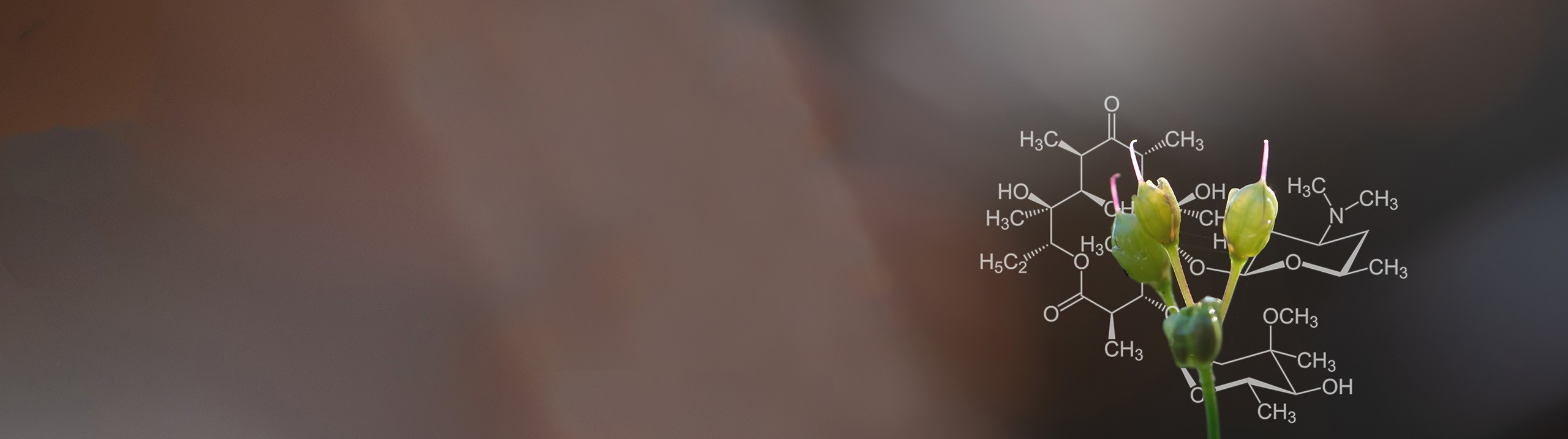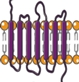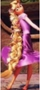Start typing, then use the up and down arrows to select an option from the list.# Biochemistry

Learn the toughest concepts covered in Biochemistry with step-by-step video tutorials and practice problems by world-class tutors

4. Protein Structure

# Alpha Helix Pitch and Rise

1

#### concept

Alpha Helix Pitch and Rise3m
Play a video:
in this video, we're going to talk about the Alfa Helix pitch and rise, So both the Alfa Helix pitch and rise are referring to a specific length or distance along the Alfa Helix axis. And so the Alfa Helix pitch is literally just the length or distance per turn along the Alfa helix axis between adjacent corresponding points. And because the pitches literally just the length or distance, it's measured in units of distance and the pitches literally equal to 5.4 Angstrom and Angstrom is a unit of distance symbolized with the symbol shown here and so down below. In our example, you can see that one angstrom is equivalent to 10 to the negative 10 m. And because the pitches literally the length or distance per turn, the pitch literally indicates one single turn of the Alfa Helix backbone. And in one single turn of the Alfa Helix backbone, there are 3.6 amino acid residues, and so taking a look down below at our example, you'll see we have a backbone in an Alfa helix confirmation, and you can tell because the backbone is coiling up and making these spiral like confirmation where it's making these 360 degree rotations of the backbone. And so notice that the are groups of the backbone are completely excluded in this image so that we can Onley focus on the backbone and notice that this white poll that's going through the center here is the Alfa Helix axis. And so literally the pitch is a specific distance along the Alfa Helix axis that corresponds toe one turn of the backbone so you can see that the pitch is literally referring to the distance between these two adjacent corresponding points. And that's essentially indicating one single turn of the backbone, which is 360 degrees of rotation of the backbone. And then in one turn, we know that there are 3.6 amino acid residues, as we indicated up above. And so the Alfa Helix rise is also a specific length or distance along the Alfa Helix axis. But it's not the length or distance per turn. It's the length or distance covered per amino acid residue. And so, in order to get the rise, which is the distance para amino acid residue, we take the pitch, which is the length along the axis per turn, divided by the number of residues, and that will give us the rise. So essentially, if you take 5.4 and divided by 3.6, you'll get the rise, which is 1.5 and so down below. In our image, we could indicate that the rise is 1.5. And again, that's the distance between, uh, or the distance covered along the axis for one amino acid residue. And because in one turn there are 360 degrees of rotation and also in one turn, there are 3.6 amino acid residues, so 3.6 residues. Essentially, if you take 360 divided by 3.6, you'll get an answer of 100 degrees, which says that there's 100 degrees of rotation of the backbone per residue. And that's exactly what this next point down here, saying essentially that the Alfa Helix backbone turned ah, 100 degrees per residue. And that's why we see that the rise is associated with 100 degrees of rotation of the backbone. And so in our next lesson video, we'll talk about how we can use the Alfa Helix pitch and rise to calculate the length of an Alfa helix. And so that concludes our lesson on the Alfa Helix. Pitch and rise, and I'll see you guys in our next video.
2
Problem

How many amino acid residues are needed for the α-helix backbone to obtain exactly one full periodic repeat?

3

#### concept

Alpha Helix Pitch and Rise1m
Play a video:
So now that we know about the Alfa Helix pitch and rise, we can talk about how to calculate the length of an Alfa helix. And so the length of an Alfa helix can actually be pretty easily calculated. All you really need to know is the total number of amino acid residues, and you need to be able to remember the Alfa Helix rise, which remember that the rise is the length per residue, and that's that small distance of just 15 angstrom. So down below we have the equation for calculating the length of an Alfa helix. And again, it's just gonna be the total number of amino acid residues multiplied by the Alfa Helix rise, which again is just 1.5 angstrom and so down below. In our example, it's asking us what is the length of an Alfa helix containing 52 amino acids. And so, if we were to draw our Alfa Helix here, let's say this is our Alfa Helix. Uh, basically, it's saying that from this point to this point over here that there are 52 amino acids, A and so for the length. If we're calculating the length all we need to know is the total number of amino acid residues in the Alfa Helix, which is 52. So 52 amino acids and we need to multiply this by our rise and our rise is just 1.5 angstrom. And so if we type this into our calculator 52 times 1.5, what comes out is 78 Angstrom. And so this matches up with answer option D, so we could go ahead, give d a check mark to indicate D is correct, and all our other ones here are incorrect and we can cross him off. So it's a pretty easy formula. Take the number of residues multiplied by the rise, so we'll get some practice calculating the length of an Alfa helix and our practice problems. I'll see you in those videos.
4
Problem

Suppose a cell membrane is 45 Å thick & an embedded protein has 7 parallel transmembrane α-helical segments. Calculate the minimum # of aa-residues required for all 7 α-helical segments to traverse the membrane.5
Problem

Hair is predominantly made of α-helix structures. Suppose hair grows at a rate of 20 cm/year. What is the rate at which amino acid residues are synthesized to account for the indicated growth of hair?